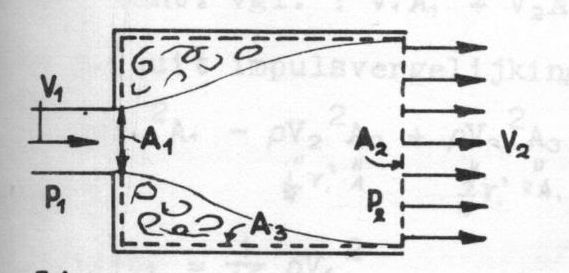## Differential equations solveable independently of coordinate system?

Integral solutions of differential equations, don't they count as coordinate free solutions?
Plenty examples of these are found in e.g. Fluid Dynamics. Here is a picture from an old textbook, as an illustration of how it works.First define a closed control volume as indicated in the picture (dotted line). Assumptions are: incompressible fluid, uniform velocity distribution over $A_1$ and $A_2$, friction along $A_3$ negligible.
Known quantities: pressure $p_1$, areas $A_1$, $A_2$, velocity $V_1$. Then $V_2$ follows from integrating the continuity (partial differential) equation over the control volume, giving: $$V_2 A_2 = V_1 A_1$$ Next find $(p_2 - p_1)$ from integrating the momentum (partial differential) equation in $x$-direction, if written as in the book: $$\iint_A \rho u (\vec{v}\cdot\vec{n}) df = - \iint_A p \cos(n,x) df$$ Giving: $$- \rho V_1^2 A_1 + \rho V_2^2 A_2 = p_1 A_2 - p_2 A_2$$ Combining this with the continuity equation gives the unknown pressure $p_2$ as: $$p_2 = p_1 + \rho V_2 (V_1 - V_2)$$ There are plenty examples as well with the integral (instead of the differential) formulations of Electromagnetism.